3

前言

1.輸入
2.權重.偏權重
3.活化函數
4.重複2 ~ 3步驟(依網路深度)
5.輸出函數
6.損失函數
7.計算梯度，數值微分(偏微分)
8.訓練權重(使用梯度下降)
9.重複2 ~ 8步驟(依訓練次數等等)

正向傳播

f(x)對W1的偏微分(變化)，帶入上次提到的數值微分得到x。
f(x)對b1的偏微分(變化)，帶入上次提到的數值微分得到1。

• 第一層f(x) = W1 * x + b1。
• 第二層g(x) = W2 * x + b2。
• 第三層h(x) = W3 * x + b3。
這時候我們計算神經網路時的公式會是h(g(f(x)))，一樣使用數值微分，則要計算。
h(g(f(x)))對W3的偏微分(變化)，h(g(f(x)))對b3的偏微分(變化)。
h(g(f(x)))對W2的偏微分(變化)，h(g(f(x)))對b2的偏微分(變化)。
h(g(f(x)))對W1的偏微分(變化)，h(g(f(x)))對b1的偏微分(變化)。

反向傳播

連鎖率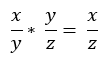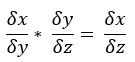反向傳播

1.dy * h(x3)對W3的偏微分(變化) = dW3，dy * h(x3)對b3的偏微分(變化) = db3，dy * h(x)對x的偏微分(變化) = dx3。(圖二)

2.dx3 * g(x2)對W2的偏微分(變化) = dW2，dx3 * g(x2)對b2的偏微分(變化) = db2，dW2 * g(x)對x的偏微分(變化) = dx2。(圖三)

3.dx2 * f(x1)對W3的偏微分(變化) = dW1，dy * f(x1)對b1的偏微分(變化) = db1，最後的x不用在計算因為x是輸入，除非還有經過處理。(圖四)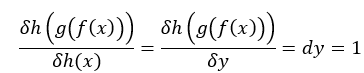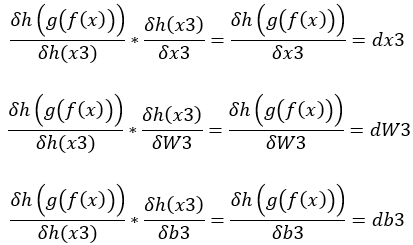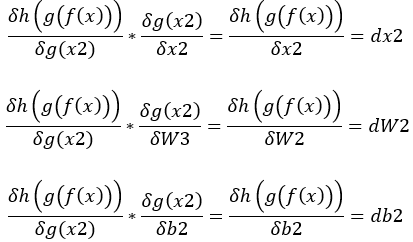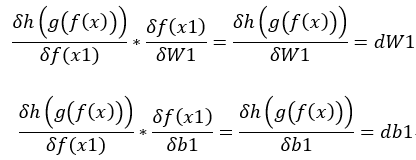權重函數

1.x * W = x1。
2.x1 + b = y。

f(x) = x * W + b =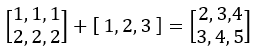x1 + b = y
1.db = dy每行加總(與d陣列大小相同即可)。
2.dx1 = dy。

x * W = x1
3.dW = 反轉x * dx1。
4.dx = dx1 * 反轉W。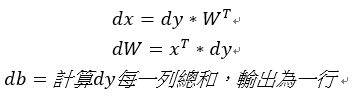活化函數Sigmoid。

1.-1 * x = x1
2.e^x1 = x2
3.1 + x2 = x3
4.1 / x3 = y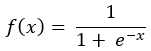1 / x3 = y
1.dx3 = -1 / x3^2

1 + x2 = x3
2.dx2 = dx3

e^x1 = x2
3.dx1 = dx2 * e^x1

-1 * x = x1
4.dx = dx1 * -1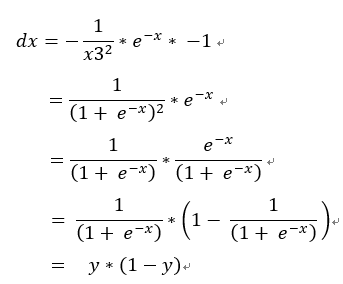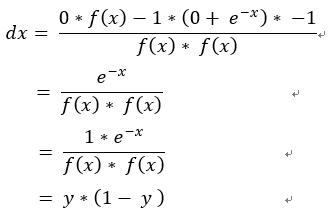輸出函數+損失函數

Softmax:
1.exp(x) = x1
2.sigma(exp(x)) = x2
3.x1 / x2 = x3

Loss Cross:
4.log(x3) = x4
5.x4 * t = x5
6.sigma(x5) = x6
7.x6 * -1 = y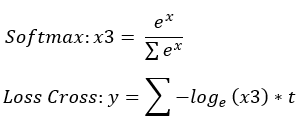Loss Cross:
x6 * -1 = y
1.dx6 = -1

sigma(x5) = x6
2.dx5 = dx6 = -1(加法直接傳遞)

x4 * t = x5
3.dx4 = dx5 * t = -t

log(x3) = x4
log(x)的微分是1 / x 證明，這裡採用的log都是e為基底，所以不用乘上ln
4.dx3 = dx4 * 1 / x3 = -t / x3

Softmax:

x1 / x2 = x1 * 1 / x2
5.d(1 / x2) = x1 * dx3 = exp(x) * -t / x3 = -t * sigma(exp(x))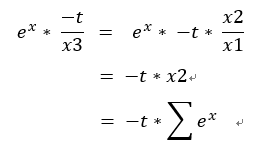6.dx1 = dx3 * (1 / x2) = -t / exp(x)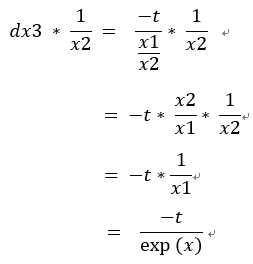1 / x2
7.dx2 = d(1 / x2) * -1 / x2^2 = 1 * sigma(exp(x))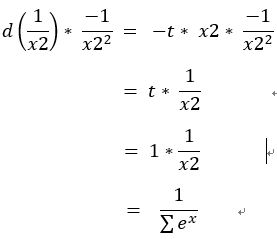sigma(exp(x)) = x2
8.d(exp(x)) = dx2 (加法直接傳遞)

exp(x)

dx = (d(exp(x)) + dx1) * exp(x) = x3 - t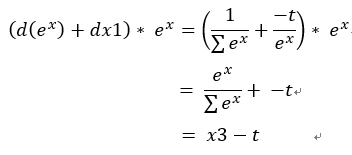結論

1.輸入
2.權重.偏權重(forward)
3.活化函數(forward)
4.重複2 ~ 3步驟(依網路深度)
5.輸出 + 損失函數(forward)
7.反向進行backward從輸出+損失開始->重複3 ~ 2(backward)，權重部分記錄dW和db才能更新權重。
8.訓練權重(使用梯度下降)
9.重複2 ~ 8步驟(依訓練次數等等)

＊數值微分用處變為拿來驗證推導的反向傳播是否正確